JEE  >  JEE Main Previous year questions (2016-20): The p-Block Elements

# JEE Main Previous year questions (2016-20): The p-Block Elements - Notes | Study Chemistry for JEE - JEE

 1 Crore+ students have signed up on EduRev. Have you?

Q.1. The electron gain enthalpy (in kJ mol–1) of fluorine, chlorine, bromine and iodine, respectively, are    (2020)
(a) −296, −325, −333 and −349
(b) −349, −333, −325 and −296
(c) −333, −349, −325 and −296
(d) −333, −325, −349 and −296

Ans. (c)

The electron gain enthalpy of halogens is given below:

 Halogens Electron Gain Enthalpy (ΔH) in kJ mol–1 F -333 CI -349 Br -325 I -296

Q.2. Chlorine reacts with hot and concentrated NaOH and produces compounds (X) and (Y). Compound (X) gives white precipitate with silver nitrate solution. The average bond order between Cl and O atoms in (Y) is ______.    (2020)

Ans. (1.67)

The reaction involved is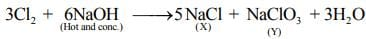The structure of NaClO3 is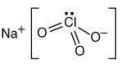Two Cl–O bonds are double bond and one is single bond, thus the average bond order between Cl and O is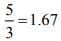Q.3. In the following reactions, products (A) and (B), respectively are:
NaOH (hot and conc.) + Cl2 → (A) + side products
Ca(OH)2 (dry) + Cl2 → (B) + side products    (2020)
(a) NaClO3 and Ca(OCl)2
(b) NaClO3 and Ca(ClO3)2
(c) NaOCl and Ca(OCl)2
(d) NaOCI and Ca(CIO
3

Ans. (a)

The reaction involved is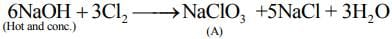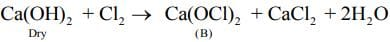Q.4. The number of bonds between sulphur and oxygen atoms in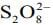and the number of bonds between sulphur and sulphur atoms in rhombic sulphur, respectively, are    (2020)
(a) 4 and 6
(b) 8 and 8
(c) 8 and 6
(d) 4 and 8

Ans. (b)

The structure ofis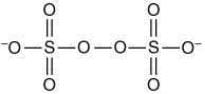There is total of 8 bonds present in between S and O.
Struture of rhombic sulphur is: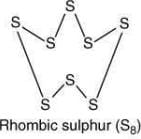There are a total of 8 S-S bonds.

Q.5. White phosphorus on reaction with concentrated NaOH solution in an inert atmosphere of CO2 gives phosphine and compound (X). (X) on acidification with HCl gives compound (Y). The basicity of compound (Y) is    (2020)
(a) 2
(b) 1
(c) 4
(d) 3

Ans. (b)

The reaction involved is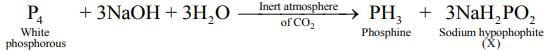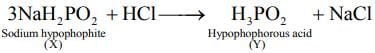The structure of the hypophosphorous acid is: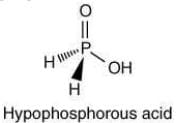Hypophosphorous acid have only one replaceable hydrogen atom, thus, its basicity is one.

Q.6. The reaction of H3N3B3Cl3(A) with LiBH4 in tetrahydrofuran gives inorganic benzene (B). Further, the reaction of (A) with (C) leads to H3N3B3(Me)3. Compounds (B) and (C) respectively, are    (2020)
(a) borazine and MeBr
(b) diborane and MeMgBr
(c) boron nitride and MeBr
(d) borazine and MeMgBr

Ans. (d)

The reaction involved is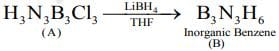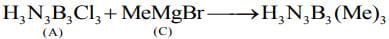Q.7. The one that is extensively used as a piezoelectric material is:    (2019)
(a) tridymite
(b) amorphous silica
(c) quartz
(d) mica

Ans. (c)
Quartz exhibits piezo electricity and thus can be used as a piezoelectric material.

Q.8. Correct statements among a to d regarding silicones are:    (2019)
(a) They are polymers with hydrophobic character.
(b) They are biocompatible.
(c) In general, they have high thermal stability and low dielectric strength.
(d) Usually, they are resistant to oxidation and used as greases.
(a) (a), (b), (c) and (d)
(b) (a), (b) and (c) only
(c) (a) and (b) only
(d) (a), (b) and (d) only

Ans. (d)

Silicones are polymers containing Si—O—Si linkages with strong hydrophobic character.
Generally, they exhibit high thermal stability with high dielectric strength. Silicon greases are resistant to oxidation which are commonly used for greasing purposes.

Q.9. Good reducing nature of H3PO2 is attributed to the presence of:    (2019)
(a) Two P — OH bonds
(b) One P — H bond
(c) Two P — H bonds
(d) One P — OH bond

Ans. (c)
Structure of H3PO2: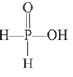Greater the number of P—H bonds present in the acid, greater will be its reducing property.

Q.10. Wilkinson catalyst is:    (2019)
(a) [(Ph3P)3 IrCl]
(b) [(Et3P)3 RhCl]
(c) [(Ph3P)3 RhCl]
(d) [(Et3P)3 IrCl]

Ans. (c)
Wilkinson’s catalyst is [Rh(PPh3)3Cl]

Q.11. The type of hybridisation and number of lone pair(s) of electrons of Xe in XeOF4, respectively, are:    (2019)
(a) sp3d2 and 1
(b) sp3d and 2
(c) sp3d2 and 2
(d) sp3d and 1

Ans. (a)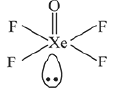, sp3d2, no. of lone pair = 1

Q.12. Among the following reactions of hydrogen with halogens, the one that requires a catalyst is:    (2019)
(a) H2 + I2 → 2 HI
(b) H2 + Cl2 → 2 HCl
(c) H2 + Br2 → 2HBr
(d) H2 + F2 → 2HF

Ans. (a)
The reaction between I2 and H2 requires catalyst, whereas all other halogens react with H2 without the requirement of a catalyst.

Q.13. The pair that contains two P-H bonds in each of the oxoacids is:    (2019)
(a) H4P2O5 and H4P2O6
(b) H3PO2 and H4P2O5
(c) H3PO3 and H3PO2
(d) H4P2O5 and H3PO3

Ans. (b)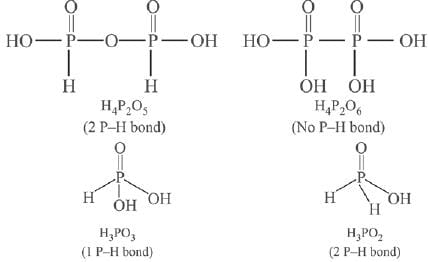Q.14. The number of 2-centre-2-electron and 3-centre-2-electron bonds in B2H6, respectively, are:    (2019)
(a) 2 and 1
(b) 4 and 2
(c) 2 and 2
(d) 2 and 4

Ans. (b)
Structure of B2H6: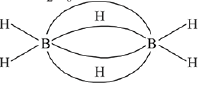∴ No. of 2-centre-2 electron bonds = 4,
No. of 3-centre-2 electron bonds = 2.

Q.15. The chloride that CANNOT get hydrolysed is:    (2019)
(a) PbCl4
(b) CCl4
(c) SnCl4
(d) SiCl4

Ans. (b)
CCl4 cannot be hydrolysed due to absence of d orbitals at carbon atom.

Q.16. The hydride that is NOT electron deficient is:    (2019)
(a) SiH4
(b) B2H6
(c) GaH3
(d) AlH3

Ans. (a)
SiH4: Electron precise hydride
B2H6, GaH3 and Al H3 are electron deficient

Q.17. Iodine reacts with concentrated HNO3 to yield Y along with other products. The oxidation state of iodine in Y, is:    (2019)
(a) 5
(b) 7
(c) 3
(d) 1

Ans. (a)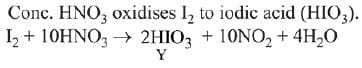In HIO3 oxidation state of iodine is +5.

Q.18. The element that does NOT show catenation is:    (2019)
(a) Ge
(b) Si
(c) Sn
(d) Pb

Ans. (d)
Catenation power of the elements decreases as we move down in the group. Therefore, Pb does not show catenation property.

Q.19. Chlorine on reaction with hot and concentrated sodium hydroxide gives:    (2019)
(a) Cl- and CIO-3
(b) Cl- and ClO-
(c) ClO-3 and ClO-2
(d) Cl- and ClO-2

Ans. (a)
3Cl+ 6 NaOH → 5 NaCl + NaClO+ 3 H2O

Q.20. The element that shows greater ability to form pπ - pπ multiple bonds, is:    (2019)
(a) Sn
(b) C
(c) Ge
(d) Si

Ans. (b)
Carbon has unique ability to form pπ-pπ multiple bonds with itself and with other atoms of small size. Heavier elements do not form pπ-pπ bonds because their atomic orbitals are too large and diffuse to have effective overlapping.

Q.21. Diborane (B2H6) reacts independently with O2 and H2O to produce, respectively:    (2019)
(a) B2O3 and H3BO
(b) B2O3 and [BH4]-
(c) H3BO3 and B2O3
(d) HBO2 and H3BO3

Ans. (a)
B2H6 + 3O2 → B2O3 + 3H2O
B2H6 + 6H2O → 2H3BO3 + 6H2

Q.22. The correct statement about ICI5 and ICI-4 is:    (2019)
(a) both are is isostructural.
(b)  ICI5 is trigonal bipyramidal and ICI-4 is tetrahedral.
(c) ICI5 is square pyramidal and ICI-4 is tetrahedral.
(d) ICI5 is square pyramidal and ICI-4 is square planar.

Ans. (d)
ICI5 is sp3d2 hybridised (5 bp, 1 lp)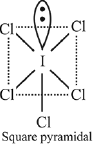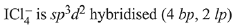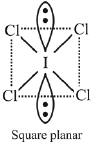Q.23. The ion that has sp3d2 hydridization for the central atom, is:    (2019)
(a) [ICI4]-
(b) [ICI2]
(c) [IF6]-
(d) [BrF2]-

Ans. (a)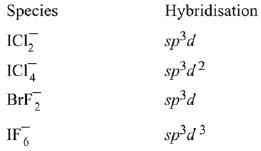Q.24. The correct order of the oxidation states of nitrogen in NO, N2O, NO2 and N2O3 is:    (2019)
(a) NO2 < NO < N2O3 < N2O
(b) NO2 < N2O3 < NO < N2O
(c) N2O < N2O3 < NO < NO2
(d) N2O < NO < N2O3 < NO2

Ans. (d)
order of oxidation state –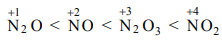Q.25. C60, an allotrope of carbon cantains:    (2019)
(a) 12 hexagons and 20 pentagons.
(b) 18 hexagons and 14 pentagons.
(c) 16 hexagons and 16 pentagons.
(d) 20 hexagons and 12 pentagons.

Ans. (d)
Fullerene (C60) contains 20 hexagons (six membered) rings and 12 pentagons (five membered rings)

Q.26. HF has highest boiling point among hydrogen halides, because it has:    (2019)
(a) strongest van der Waals’ interactions
(b) lowest ionic character
(c) strongest hydrogen bonding
(d) lowest dissociation enthalpy

Ans. (c)
Due to strong H-bonding between HF molecules. HF has highest boiling point among the hydrogen halides.

Q.27. The amorphous form of silica is:    (2019)
(a) Tridymite
(b) Kieselguhr
(c) Cristobalite
(d) Quartz

Ans. (b)
Quartz, tridymite and cristobalite are crystalline forms of silica, while kieselguhr is an amorphous form of silica.

Q.28. The oxoacid of Sulphur that does not contain bond between Sulphur atoms is:    (2019)
(a) H2S4O6
(b) H2S2O3
(c) H2S2O7
(d) H2S2O4

Ans. (c)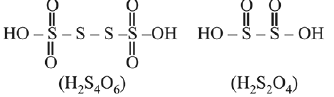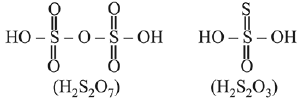H2S2O7 does not show bonding between sulphur atoms.

Q.29. The correct order of catenation is:    (2019)
(a) C > Sn > Si ≈ Ge
(b) C > Si > Ge ≈ Sn
(c) Si > Sn > C > Ge
(d) Ge > Sn > Si > C

Ans. (b)
The catenation property among 14th group elements is based on bond enthalpy value of bond between the same element. The decreasing order of bond enthalpy values is
Bond enthalpy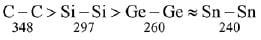in kJ/mol
∴ Decreasing order of catenation is
C > Si > Ge ≈ Sn

Q.30. The number of pentagons in C60 and trigons (triangles) in white phosphorous, respectively, are:    (2019)
(a) 20 and 3
(b) 12 and 4
(c) 12 and 3
(d) 20 and 4

Ans. (b)
Number of pentagons in C60 (Buckminsterfullerene) = 12
Number of triangles in P4 (White phosphorous) = 4

Q.31. The noble gas that does NOT occur in the atmosphere is:    (2019)
(a) He
(b) Kr
(c) Ne
(d) Ra

Ans. (d)

Q.32. The basic structural unit of feldspar, zeolites, mica, and asbestos is:    (2019)
(a) (SiO3)2-
(b) SiO2
(c) (SiO4)4-
(d)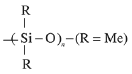Ans. (c)
These are examples of silicates, the basic unit of each of them is SiO.

Q.33. The C - C bond length is maximum in:    (2019)
(a) graphite
(b) C70
(c) C60
(d) diamond

Ans. (d)
Carbon-carbon bond length is maximum in diamond because diamond has all single bonds while graphite, C70 and C60 have single and double bonds.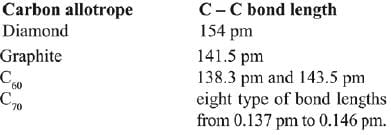Q.34. Which of the following compounds contain(s) no covalent bond(s)?    (2018)
KCl, PH3, O2, B2H6, H2SO4
(a) KCl, B2H6, PH3
(b) KCl, H2SO4
(c) KCl
(d) KCl, B2H6

Ans. (c)
KCl is an ionic compound. It cannot from covalent bond. Elements of s - block & p - block combine to form ionic compounds.

Q.35. Total number of lone pair of electrons in I-3 ion is:    (2018)
(a) 3
(b) 6
(c) 9
(d) 12

Ans. (c)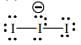Q.36. The compound that does not produce nitrogen gas by the thermal decomposition is:    (2018)
(a) Ba(N3)2
(b) (NH4)2Cr2O7
(c) NH4NO2
(d) (NH4)2SO4

Ans. (d)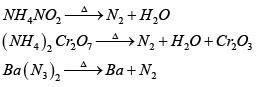Q.37. Xenon hexafluoride on partial hydrolysis produces compounds 'X' and 'Y'. Compounds 'X' and 'Y' and the oxidation state of Xe are respectively:    (2018)
(a) XeOF(+ 6) and XeO2F2 (+6)
(b) XeOF4 (+ 6) and XeO3 (+6)
(c) XeO2F2 (+ 6) and XeO2 (+4)
(d) XeO2(+ 4) and XeO3 (+6)

Ans. (a)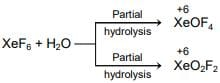Q.38.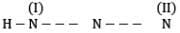In hydrogen azide (above) the bond orders of bonds (I) and (II) are:    (2018)
(a) <2, <2
(b) <2, >2
(c) >2, >2
(d) >2, <2

Ans. (b)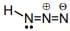Or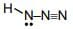Q.39. The decreasing order of bond angles in BF3, NH3, PF3 and I3- is:    (2018)
(a) BF3 > NH3 > PF3 > I3
(b) I3> BF3 > NH3 > PF3
(c) I3 > NH3 > PF3 > BF3
(d) BF3 > I3 > PF3 > NH3

Ans. (b)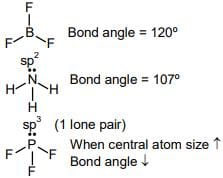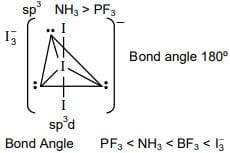Q.40. Among the oxides of nitrogen:    (2018)
N2O3, N2O4 and N2O5 ; the molecule(s) having nitrogen-nitrogen bond is/are:
(a) N2O3 and N2O4
(b) N2O4 and N2O5
(c) N2O3 and N2O5
(d) Only N2O5

Ans. (a)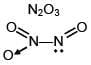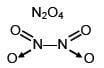Q.41. A group 13 element 'X' reacts with chlorine gas to produce a compound XCl3. XCl3 is electron deficient and easily reacts with NH3 to form Cl3X → NH3 adduct; however, XCldoes not dimerize. X is:   (2018)
(a) B
(b) Al
(c) In
(d) Ga

Ans. (a)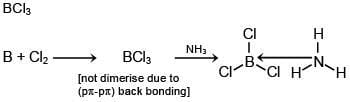Q.42. Which of the following reactions is an example of a redox reaction?    (2017)
(a) XeF4 + O2F2 → XeF6 + O2
(b) XeF2 + PF5 → [XeF]+ PF6-
(c) XeF+ H2O → XeOF4 + 2HF
(d) XeF6 + 2H2O → XeO2F2 + 4HF

Ans. (a)
Xe is oxidised from +4(in XeF4) to +6(in XeF6)
Oxygen is reduced from +1 (in O2F2) to zero (in O2)

Q.43. The products obtained when chlorine gas reacts with cold and dilute aqueous NaOH are    (2017)
(a) CIO- and CIO3-
(b) CIO2- and CIO-
(c) Cl- and CIO-
(d) Cl- and CIO2-

Ans. (c)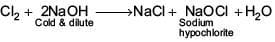Q.44. The number of S = O and S – OH bonds present in peroxodisulphuric acid and pyrosulphuric acid respectively are:    (2017)
(a) (2 and 4) and (2 and 4)
(b) (4 and 2) and (4 and 2)
(c) (4 and 2) and (2 and 4)
(d) (2 and 2) and (2 and 2)

Ans. (b)
Peroxodisulphuric acid
H2S2O8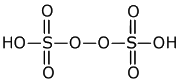Pyrosulphuric acid
H2S2O7 :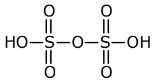Q.45. In which of the following reactions, hydrogen peroxide acts as an oxidizing agent?    (2017)
(a) HOCl + H2O2 → H3O+ + Cl- + O2
(b) I2 + H2O+ 2OH- → 2I+ 2H2O + O2
(c) PbS + 4H2O2 → PbSO4 + 4H2O
(d) 2MnO4- + 3H2O2 → 2MnO2 + 3O2 + 2H2O + 2OH-

Ans. (c)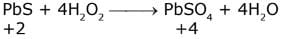Q.46. The number of P–OH bonds and the oxidation state of phosphorus atom in pyrophosphoric acid (H4P2O7) respectively are:    (2017)
(a) five and four
(b) four and five
(c) five and five
(d) four and four

Ans. (b)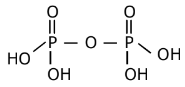Q.47. The correct sequence of decreasing number of π-bonds in the structure of H2SO3, H2SO4 and H2S2O7 is:    (2017)
(a) H2S2O7 > H2SO3 > H2SO4
(b) H2S2O7 > H2SO4 > H2SO3
(c) H2SO4 > H2S2O7 > H2SO3
(d) H2SO3 > H2SO4 > H2S2O7

Ans. (b)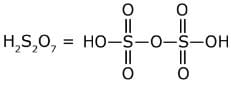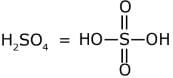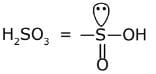Q.48. XeF6 on partial hydrolysis with water produces a compound ‘X’. The same compound ‘X’ is formed when XeF6 reacts with silica. The compound ‘X’ is:    (2017)
(a) XeF4
(b) XeF2
(c) XeO3
(d) XeOF4

Ans. (d)

Q.49. The pair in which phosphorous atoms have a formal oxidation state of + 3 is:    (2016)
(a) Orthophosphorous and pyrophosphorous acids
(b) Pyrophosphorous and hypophosphoric acids
(c) Orthophosphorous and hypophosphoric acids
(d) Pyrophosphorous and pyrophosphoric acids

Ans. (a)
Phosphorus acid series contain phosphorus in the oxidation state (+ III).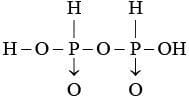Pyrophosphorus acid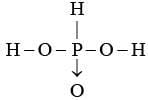orthophosphorus acid

Q.50. The non-metal that does not exhibit positive oxidation state is:    (2016)
(a) Fluorine
(b) Oxygen
(c) Chlorine
(d) Iodine

Ans. (a)
Fluorine is the most electronegative element in periodic table hence it shows –1 oxidation state in all its compounds.

Q.51. The group of molecules having identical shape is:    (2016)
(a)  PCl5, IF5, XeO2F2
(b) BF3, PCl3, XeO3
(c)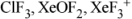(d) SF4, XeF4, CCl4

Ans. (c)are sp3d hybridized with 2 lone pair e's, hence all have (T-shape) identical shape.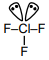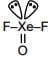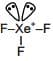Q.52. Which intermolecular force is most responsible in allowing xenon gas to liquefy?    (2016)
(a) Instantaneous dipole-induced dipole
(b) Ionic
(c) Ion-dipole
(d) Dipole-dipole

Ans. (a)
Instantaneous dipole-induced dipole forces are most responsible in allowing xenon gas to liquify.

The document JEE Main Previous year questions (2016-20): The p-Block Elements - Notes | Study Chemistry for JEE - JEE is a part of the JEE Course Chemistry for JEE.
All you need of JEE at this link: JEE

## Chemistry for JEE

322 videos|586 docs|368 tests
 Use Code STAYHOME200 and get INR 200 additional OFF

## Chemistry for JEE

322 videos|586 docs|368 tests

Track your progress, build streaks, highlight & save important lessons and more!

,

,

,

,

,

,

,

,

,

,

,

,

,

,

,

,

,

,

,

,

,

;